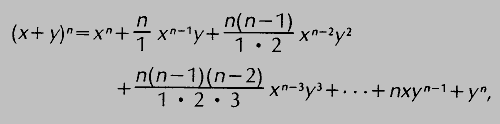binomial

(redirected from binomial population)
Also found in: Dictionary, Thesaurus, Medical, Financial.

binomial

(bī'nō`mēəl), polynomial expression (see polynomialpolynomial,
mathematical expression which is a finite sum, each term being a constant times a product of one or more variables raised to powers. With only one variable the general form of a polynomial is a0xn+a1x
) containing two terms, for example, x+y. The binomial theorem, or binomial formula, gives the expansion of the nth power of a binomial (x+y) for n=1, 2, 3, … , as follows:where the ellipsis (…) indicates a continuation of terms following the same pattern. For example, using the formula and reducing fractions, one obtains (x+y)5=x5+5x4y+10x3y2+10x2y3+5xy4+y5. The coefficients 1, n, n (n−1)/1·2, etc., of x and y may also be found from an array known as Pascal's triangle (for Blaise PascalPascal, Blaise
, 1623–62, French scientist and religious philosopher. Studying under the direction of his father, a civil servant, Pascal showed great precocity, especially in mathematics and science.
), formed by adding adjacent numbers to find the number below them as follows:binomial

[bī′nō·mē·əl]
(mathematics)
A polynomial with only two terms.
McGraw-Hill Dictionary of Scientific & Technical Terms, 6E, Copyright © 2003 by The McGraw-Hill Companies, Inc.

binomial

1. a mathematical expression consisting of two terms, such as 3x + 2y
2. a two-part taxonomic name for an animal or plant
3. referring to two names or terms
Collins Discovery Encyclopedia, 1st edition © HarperCollins Publishers 2005
Site: Follow: Share:
Open / Close